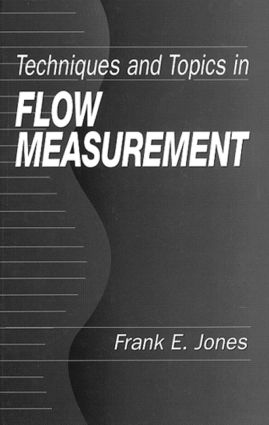Techniques and Topics in Flow Measurement

1st Edition

CRC Press

176 pages

Purchasing Options:\$ = USD
Hardback: 9780849324758
pub: 1995-08-08
SAVE ~\$37.00
\$185.00
\$148.00
x

FREE Standard Shipping!

Description

Techniques and Topics in Flow Measurement covers the applications and techniques of flow measurement. This definitive book provides guidelines for choosing appropriate techniques and assuring valid measurements as well as describes methods for treatment of calibration data in fluid flow under various conditions. The book also covers three systems of units: the SI system, the English Absolute Dimensional system, and the English Engineering system. Commonly used - and often misused - variables such as force, weight, and pressure are defined, and the relationships between the systems for these common variables are summarized.

One of the many unique features of Techniques and Topics in Flow Measurement is the number of ready-to-use tables included throughout the text. Tables are provided for such commonly encountered variables as the saturation vapor pressure of water; the composition of dry air; the compressibility factor for air; air-free and air-saturated water density; viscosity of dry air, nitrogen, and other gases; and specific heat/specific volume ratios for dry air, water vapor, and moist air. Another unique feature of this book is the number of highly relevant examples. The author includes examples/exercises that demonstrate applications for density calculations; water vapor mixing ratio determination; gas viscosity interpolation; NIST guideline applications; buoyancy corrections; and more.

Force, Weight, and Pressure Units

Force

Weighing

Pressure

Application to Manometry

Density of Gases

Development of the Air Density Equation

Parameters of the Air Density Equation

Uncertainties in Air Density

Uncertainties in the Environmental Quantities

Use of Constant Values of f, Z, and Ma in the Air Density Equation

CIPM-81 Air Density Equation

Densities of Some Other Gases

Density of Water

Formulations of Kell

New Formulations

Tables

Water Vapor Mixing Ratio as a Flow Parameter

Density of Moist Air. Freestream Air Velocity

Real-Gas Ratio of Specific Heats, g, for Moist Air

Calculation of Saturation Mixing Ratio

Uncertainty in the Calculation of rs

Viscosity of Gases

Experimental Data

Development of Equations

Ranges of Application of the Equations

Tables

Gamma, Ratio of Specific Heats for Air

Real-Gas Specific Heats of Air

Real-Gas Specific Heats of Water Vapor

Measurement Uncertainty

NIST Guidelines

Buoyancy Corrections in Weighing

Buoyant Force and Buoyancy Correction

Application of the Simple Buoyancy Correction Factor to Weighing on a Single-Pan Balance

The Electronic Analytical Balance

Examples of Effects of Failure to Make Buoyancy Corrections

Real-Gas Critical Flow Factor, C*

Interpolation Formula for C*

Subsonic Flow and Discharge Coefficients

Subsonic Flow of Gas through Venturis, Nozzles, and Orifices

Nozzle Discharge Coefficient

Derivation of Discharge Coefficient, CD, for Orifice Plates

Automatic Pipets

Calibration of Automatic Pipets

Results and Conclusions

Reference Method for Testing Hydrometers

Hydrometer Calibration Method

Application of the Method to Hydrometer Calibration

Treatment of Laminar Flowmeter Calibration Data

Development of the Equation

Effect of Kinematic Viscosity on Performance of Turbine Flowmeters

Application of Dimensional Analysis

Curvature of a Plot of f/Q against f

Experimental Determinations of a(v) and b(v)

Discussion and Conclusions

Analysis of Calibration Data for Vortex Shedding Flowmeter

Treatment of Calibration Data for Venturi Meters

Ascertaining Linear Range of Anemometers

Jones's Treatment of Calibration Data for Turbine Flowmeters

Treatment of Anemometer Calibration Data

Application of the Analysis

Diverter Corrections

Diverter Valve Correction

Conclusions

Calibration of Platform Scale

Experimental

Volumetric Test Measures: Types of Test Measures

Calibration of Test Measures

Equivalence of Gravimetric and Volumetric Test Measure. Example of Uncertainty for Gravimetric Calibration

Experimental Determination of Density of Liquids

Liquid Density Determination by Hydrostatic Weighing

Determination of the Density of Liquids Using a Mechanical Oscillator Technique

Tank Volume

Tank Calibration

Calibration Equation

Application to Flow Measurement

Index